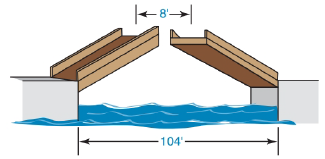Chapter 5.4, Problem 19EElementary Geometry For College St...

7th Edition
Alexander + 2 others
ISBN: 9781337614085

Solutions

Chapter
SectionElementary Geometry For College St...

7th Edition
Alexander + 2 others
ISBN: 9781337614085
Textbook Problem

A drawbridge that is 104 ft in length is raised at its midpoint so that the uppermost points are 8 ft apart. How far has each of the midsections been raised?To determine

To Find:

Length of the midsections been raised.

Explanation

Given A drawbridge that is 104 ft in length is raised at its midpoint so that the uppermost points are 8 ft apart.

The figure can be redrawn as,

From the given data,

AB = 104 ft.

CD = EF = 8 ft.

And from the redrawn figure,

AE = FB

AC=12(AB)    =12(104)    =52

And from the figure,

AB = AE+EF+FB

104 = AE+8+AE

104 = 2AE+8

2AE = 96

AE = 962

AE = 48

Still sussing out bartleby?

Check out a sample textbook solution.

See a sample solution

The Solution to Your Study Problems

Bartleby provides explanations to thousands of textbook problems written by our experts, many with advanced degrees!

Get Started

Evaluate the limit, if it exists. limx4x2+95x+4

Single Variable Calculus: Early Transcendentals, Volume I

Let f(x)={2x+1if1x0x2+2if0x2 Find (a) f(1), (b) f(0), and (c) f(32).

Applied Calculus for the Managerial, Life, and Social Sciences: A Brief Approach

In Exercise 11-14, factor the expression. y27y+494

Calculus: An Applied Approach (MindTap Course List)

Calculate y'. 35. y = cot(3x2 + 5)

Single Variable Calculus: Early Transcendentals

Explain the difference between passive and active deception.

Research Methods for the Behavioral Sciences (MindTap Course List)

Express 2.0276 meters as centimeters.

Mathematics For Machine Technology